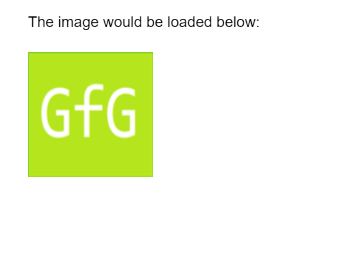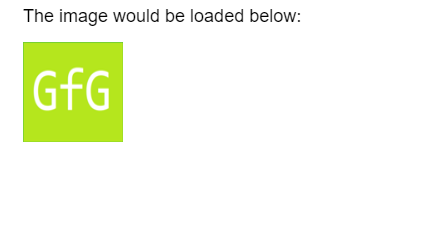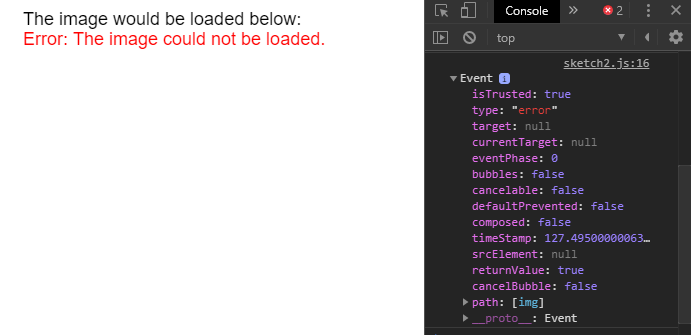Open In App
Related Articles
• Write an Interview Experience
• p5.js
• p5.js Introduction

The loadImage() function is used to load an image from the given path and create a p5.Image object with the image.

It is advised to load an image in the preload() function as the loaded image may not be available for use immediately. The image is preferably loaded from a relative path as some browsers may prevent loading from other remote locations due to the browser’s security features.

Syntax:

`loadImage(path, successCallback, failureCallback)`

Parameters: This function accepts three parameter as mentioned above and described below.

• path: This is the path from where the image is to be loaded.
• successCallback: This is a function which is called if the image successfully loads and it is an optional parameter.
• failureCallback: This is a function which is called if the image does not load due to any error and it is an optional parameter.

Below examples illustrates the loadImage() function in p5.js:

 `let img;`` ` `function` `preload() {``  ``img = loadImage(``'sample-image.png'``);``}`` ` `function` `setup() {``  ``createCanvas(300, 200);`` ` `  ``text(``"The image would be loaded below:"``, 20, 20);``  ``image(img, 20, 40, 100, 100);``}`

Output:Example 2: This example shows loading an image from a URL and both callbacks that may take place.

 `let img;``let url = ``'https://media.geeksforgeeks.org/wp-content/uploads/20190314004249/sample-image.png'``;`` ` `function` `setup() {``  ``createCanvas(400, 200);`` ` `  ``textSize(18)``  ``text(``"The image would be loaded below:"``, 20, 20);`` ` `  ``loadImage(url, img => {``    ``image(img, 20, 40, 100, 100);``  ``},``    ``(event) => {``      ``fill(``"red"``)``      ``text(``"Error: The image could not be loaded."``, 20, 40);``      ``console.log(event);``    ``}``  ``);``}`

Output: